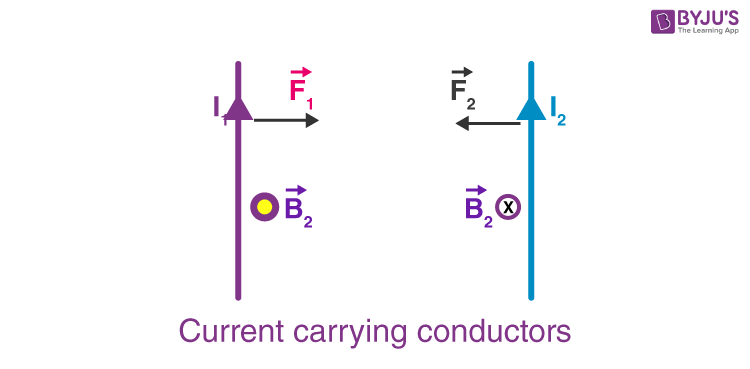# Force between two parallel Current carrying conductor

• We have learned about the existence of a magnetic field due to a current-carrying conductor and the Biot – Savart’s law.
• We have also learned that an external magnetic field exerts a force on a current-carrying conductor and the Lorentz force formula that governs this principle.
• Thus, from the two studies, we can say that any two current carrying conductors when placed near each other, will exert a magnetic force on each other. In this section, we will learn about this case in further details.Consider the system shown in the figure above. Here, we have two parallel current carrying conductor, separated by a distance ‘d’, such that one of the conductors is carrying a current I1 and the other is carrying I2, as shown in the figure. From the knowledge gained before, we can say that the conductor 2 experiences the same magnetic field at every point along its length due to the conductor 1. The direction of magnetic force is indicated in the figure and is found using the right-hand thumb rule. The direction of the magnetic field, as we can see, is downwards due to the first conductor.

From the Ampere’s circuital law, the magnitude of  the field due to the first conductor can be given by,

$$B_{a}=\frac{\mu _{0}I_{1}}{2\pi d}$$

The force on a segment of length L of the conductor 2 due to the conductor 1 can be given as,

$$F_{21}=I_{2}LB_{1}=\frac{\mu _{0}I_{1}I_{2}}{2\pi d}L$$

Similarly, we can calculate the force exerted by the conductor 2 on the conductor 1. We see that, the conductor 1 experiences the same force due to the conductor 2 but the direction is opposite. Thus,

F12 = F21

We also observe that, the currents flowing in the same direction make the conductors attract each other and that showing in the opposite direction makes the conductors repel each other. The magnitude of force acting per unit length can be given as,

$$f_{ba}=\frac{\mu _{0}I_{a}I_{b}}{2\pi d}$$

Stay tuned with BYJU’S to learn more about the force between two parallel current-carrying conductors, Magnetic Field Due To Current Carrying Conductor and much more.

Test your Knowledge on Parallel Current Carrying Conductor

#### 1 Comment

1. I love it🥰🥰🥰🥰🥰🥰❤❤❤